8.2: Trigonometric Ratios of special angles:

Since one angle in a right angled triangle is 900 the sum of other two angles has to be 900

The special pair of acute angles are (600 300 ) and(450 ,450 )

Let us study the properties of ratios in such cases

1. The special pair of (450 ,450):

 In the adjacent figure, let A = 450 hence C = 450. (sum of  two angles has to be 900) Therefore ABC is equilateral triangle with AB=BC. Let AB =a By Pythagoras theorem   AC2 = AD2+DC2 = 2a2AC =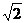a   By definition sin A = sin 45 = Opposite  Side /Hyp. =BC/AC =a/a = 1/cos A = cos 45 =Adjacent  Side /Hyp.  = AB/AC =a/a = 1/tan A = tan 45 =Opposite  Side /Adjacent  Side  =BC/AB = a/a =1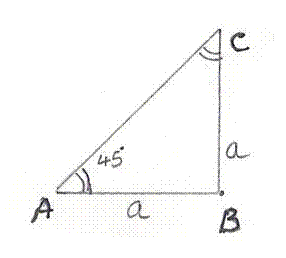2. The special pair of (600 ,300):

 Let us consider an isosceles triangle whose sides are 2a. Let CD be perpendicular  to AB Since ABC is an isosceles triangle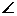A =B=C=600(all angles are equal) andACD = 300(sum of all angles in triangle ADC = 1800) By Pythagoras theorem  AC2 = AD2+DC2DC2 = AC2-AD2  = (2a)2-a2  = 3a2CD =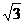a By definition sin A = sin 60 =  O/H= CD/AC =a/2a =/2 cos A = cos 60 = B/H= AD/AC =a/2a = 1/2 tan A = tan 60 = O/A =CD/AD =a/a =By definition sin ACD = sin 30=  O/H= AD/AC =a/2a = 1/2 cos ACD = cos 30= A/H= CD/AC =a/2a =/2 tan ACD  = tan 30= O/A = AD/CD = a/a =1/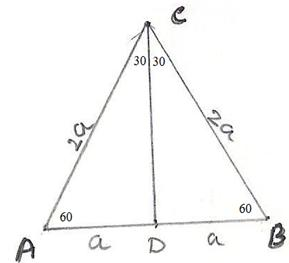3. The special pair of (00 ,900):

 When angle A approaches 900 (hypotenuse becomes Opposite  Side ) the length of Opposite  Side  and hypotenuse become same and length of Adjacent  Side  becomes zero. Thus sin 90 =  O/H= 1,  cos90= A/H =0 and tan90 = O/A = Opposite  Side/0=undefined When angle A approaches 00 (hypotenuse becomes Adjacent  Side  itself) the length of Adjacent  Side  and hypotenuse become same and Opposite  Side  becomes zero. Thus sin 0 =  O/H= 0 ,  cos0= A/H =1 and tan 0 = O/A = 0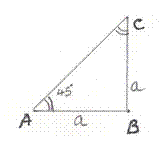The ratios for few special angles can be summarized in a table as given below:

 Angle => 00 300 450 600 900 Ratios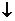Values for the anglessin(Angle) = 0 1/2 1//2 1 cos(Angle) = 1/2 1/1/2 0 tan(Angle) = 0 1/1undefined cosec(Angle) = undefined 22/1 sec(Angle) = 1 2/2 undefined cot(Angle) = undefined1 1/0

 For values of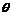= 0, 300,450,600 and 900 let us draw the graph for sin, cos and tan. First graph has values for both sin and cos represented by blue line and green line respectively.   The second graph is for tan.   Observations:   1. with the increase in angles value of sin increases from 0 to 1 2. with the increase in angles value of cos decreases from 1 to 0 3. with the increase in angles, value of tan increases from 0 to infinity. Graph of sin()  and cos() Graph for tan()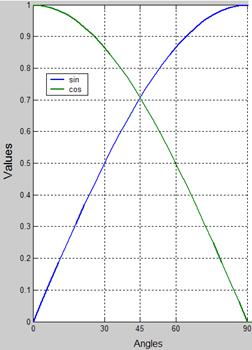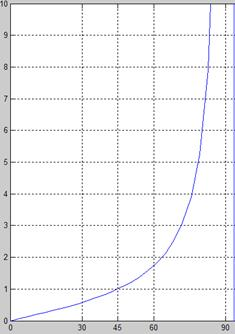Trigonometric table:

 The values of sin, cos and tan for different angles (1 to 890) are found using a table, part of which is given below The section of sine table for values from 1 to 100 and part of the degrees is given below: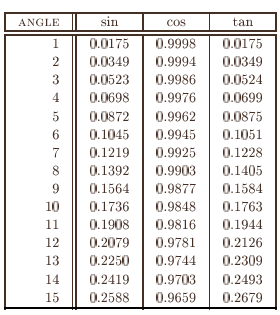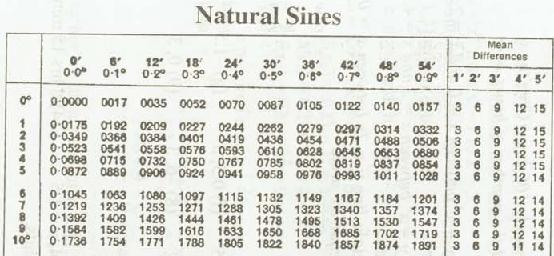8.2 Problem 1: For any angle prove

1. sin2A+ cos2A =1

2. sec2A-tan2A =1

3. cosec2A-cot2A =1

By definitions

Exercise : By substituting A = 300, 450, 600 and corresponding values for sin, cos, sec, tan, cosec, cot observe that equations in Problem 8.2.1 are true.

8.2 Problem 2:  If A and B are two acute angles in a right angled triangle prove that

sin(A+B) =1= sinAcosB+cosAsinB and cos(A+B) =0= cosAcosB-sinAsinB

Solution:

 1. sin(A+B) = sinAcosB+cosAsinB 2. cos(A+B) = cosAcosB-sinAsinB   By definition sinAcosB+CosAsinB = (BC/AB)*(BC/AB) + (AC/AB)*(AC/AB) BC2/ AB2+AC2 /AB2 = (BC2+AC2)/AB2 =1(By Pythagoras theorem) Since A and B are acute angles of the triangle, A+B = 900 Thus sin(A+B) = sin 90 = 1 This proves the first statement   Similarly the other statement can be proved.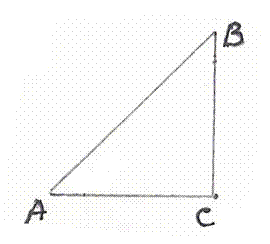Exercise : Verify the statements in 8.2 Problem 2 when (A,B) = (600 ,300 ), (300 ,600 ), (00 ,900 ) , , ,using the values in the table for  special angles.

8.2 Problem 3:  If A = 300 then prove that

cos 2A =  cos2A - sin2A = (1-tan2A)/(1+ tan2A)

Solution:

Since A = 300, 2A = 600cos 2A =cos 60 = 1/2                              -----à(1)

cos2 A = (cosA)2= (cos30)2= (/2)2 =3/4

sin2 A= (sin30)2= (1/2)2 =1/4cos2A - sin2A = 3/4 -1/4  = 1/2                  -----à(2)

tan2A = (tan 30)2= (1/)2 =1/3(1-tan2A)/(1+ tan2A) = (1-1/3)/(1+1/3)

= (2/3)/(4/3) = 2/4 = 1/2                            ------à(3)

From (1), (2) and (3) we conclude that cos 2A = cos2A - sin2A = (1-tan2A)/(1+ tan2A)

8.2 Problem 4: Find the magnitude of angle A if

2sin Acos A –cos A-2sinA+1=0

Solution:

2sin Acos A –cos A-2sinA+1 =0

cos A(2sinA-1) –(2sinA-1)=0

(2sinA-1)(cos A-1)=0(2sinA-1) =0 Or (cos A-1)=0

I.e. sin A =1/2 Or Cos A =1A=30 Or A=0 (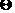sin 300 =1/2, Cos 00 =1)

Verification:

Let A =30 then

2sin Acos A –cos A-2sinA+1 = 2sin30cos30 –cos30 -2sin30+1

= 2*(1/2)* (/2) – (/2) -2*(1/2) +1

= 1*(/2) - (/2) -1+1

= (/2) - (/2) +0

=0

This proves that A=30 is one of the solution. Similarly verify that A=0 also satisfies the relationship.

8.2 Problem 5: Solve sin2 60+ cos2 (3x-9) =1

Solution:

The given equation can be rewritten as cos2 (3x-9) =1- sin2 60

Since sin 60= (/2)

sin2 60 = 3/4

Substituting this value in the given equation we get

cos2 (3x-9) =1-3/4 =1/4 = (1/2)2

cos(3x-9)  =1/2

Since 1/2 =cos 603x-9 =60

i.e. 3x =60+9=69

Therefore x =23

(Note:  we can also use the property : sin2A+ cos2A =1  )

Verification:

By substituting x=23 in cos2 (3x-9)

We get cos2 (3x-9) = cos2 (69-9) = cos2 (60) = (cos60)2 = (1/2)2= 1/4sin2 60+cos2 (3x-9)=(/2)2+1/4=3/4+1/4 = 4/4 =1 which is RHS of the given equation.

8.2 Problem 5: A point outside a circle of radius 2cm is to be chosen such that the angle between two tangents from this point to the circle is 400. How far away from the centre of the circle should this point be, if sin 20 = 0.342

 Hint: Draw a rough diagram as in the adjacent figure. 1. Join OA and OP 2. Note OP bisectsAPB andOAP =900(Refer section 6.14 Theorems) 3. Use value for sin20 (=0.342) from sine table to get the length of PO.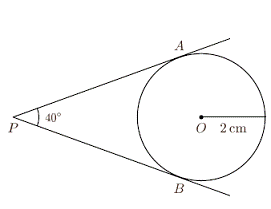8.2 Summary of learning

 No Points studied 1 Values of sin, cos, tan  and others for standard angles of 300,450,600